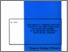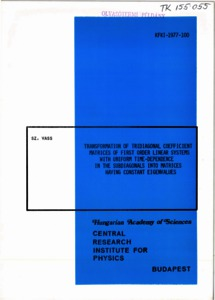# Transformation of tridiagonal coefficient matrices of first order linear systems with uniform time-dependence in the subdiagonals into matrices having constant eigenvalues

Vass, Szabolcs (1977) Transformation of tridiagonal coefficient matrices of first order linear systems with uniform time-dependence in the subdiagonals into matrices having constant eigenvalues. KFKI Reports (77-100). MTA KFKI.Preview
Text
KFKIreports_77-100.pdfEdit Item